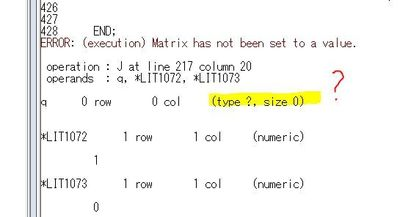## ERROR: (execution) Matrix has not been set to a value.

Hello

I keep on getting the following "ERROR: (execution) Matrix has not been set to a value." my understanding is that "q" is undefined but it is well defined!!

seems that I don't understand what is the issue exactly? I need your help, please !!!

Thank you6 REPLIES 6

## Re: ERROR: (execution) Matrix has not been set to a value.

The log tells you the line that contains the error and what the error is. On Line 217  (which is X_1_q=J(q,1,0);) the value q is undefined. The J function requires that the number of rows and columns be positive.

Notice that the loop that defines q only assigns a value if a certain logical condition is satisfied. Otherwise, q is not defined.

DO idx=1 to m-1;
if (( X_p[idx] < T) & (X_p[idx+1] >= T)) then q=idx;  /* <== not defnd if condition false */
END;

## Re: ERROR: (execution) Matrix has not been set to a value.

But the other condition is there

Do idx=1 to m-1;

if (( X[idx] < T) & (X[idx+1] >= T)) then q=idx;
end;

if ( X[m] <= T) then q= m;

with this I exhausted all the cases

## Re: ERROR: (execution) Matrix has not been set to a value.

No. For example, the situation T=0 and x={1, 2, 3} is not covered by your logic.

By the way, if you are trying to find the interval in which T is contained, then consider using the BIN function:

q = bin(T, x);

## Re: ERROR: (execution) Matrix has not been set to a value.

T in my case represents the terminal time (time to finish the study) while X represents the failure times (X1,...,Xn).  I am looking for  m failures. So either I get these m failures before the time T (that is why I have the condition T>= Xm) if not then T is between two times. That is why the ( Bin ) suggestion won't work in my case.

## Re: ERROR: (execution) Matrix has not been set to a value.

It's your program, so feel free to ignore my suggestions. But I'd like to point out that you can still use the BIN function:

q = bin(T, x);

if q = . then q=m;  /* if T out of range, assign q manually */

Anyway, good luck with your problem.

## Re: ERROR: (execution) Matrix has not been set to a value.

I am so so grateful to your help.  I didn't know that I still can use (Bin) which is why I was trying to explain my problem in more detail.

Thank you again for your help. Iam definitely going to take your suggestion.

From The DO Loop